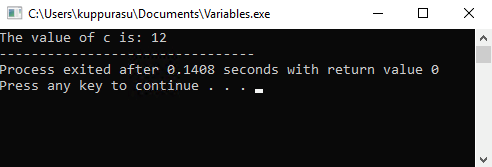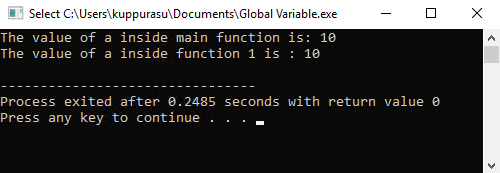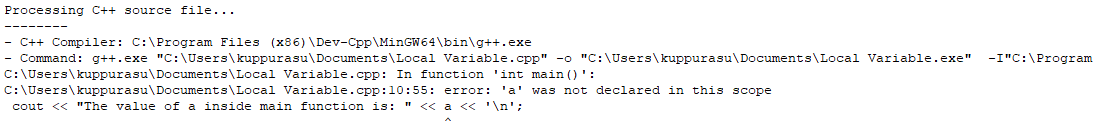# C++ Variables and Scope

22 Aug 2022
Beginner
3.66K Views

Variables in C++ are basically used to store any value. You can define a variable in different ways with different memory requirements.For example, when we write the code int a = 5, the compiler will allocate a memory location with the name ‘a’ (of data type integer) and store the value ‘5’ in the RAM.

Variables can be defined with the following data types :

• Bool

• Char

• Int

• Float

• Double

• Void

### Variable Definition

Variable definition means to tell the compiler how much space to create for the variable. The data type next to the variable will tell the compiler how much space to allocate for the variable.

The syntax for variable definition is :
```
datatype variable;
```
Examples of variable definition
```
int a;
char a, b, c;
float x, y;
```

### Variable Declaration and Initialization

Once you have defined the variable, you can assign a value to the variable.

The syntax for variable definition is :
```
datatype variable;
variable = value;
```
Example of variable declaration and initialization
```
int a;
a=1;
```

### Variable Definition, Declaration and Initialization in a single step

You can perform the variable declaration and initialization in the same step as shown below :

```
int a=1;
int a=1 , b=2;
```

#### Example Program

```
#include <iostream>
using namespace std;

int main ()
{
int a;
int b=2;
int c;
a = 10;
c = a + b;
cout << "The value of c is: " << c;
}
```

The output of this program will be :## Rules for declaring a variable in C++

When declaring a variable in C++, you must keep the following points in mind :

1. Variable names should start with an alphabet or underscore (_). The alphabet can be either A-Z (uppercase) or a-z (lowercase)

2. Variable names can contain alphabets, numbers, underscore. The alphabets can be either A-Z or a-z, numbers can be between 0-9. You cannot use any other special character other than the underscore (_) such as @,#,\$,%,&.

3. Variables can be of any length. However, it is recommended to have only a maximum of 31 characters for effective portability to any environment.

4. Reserved C++ keywords cannot be used as a variable name. Example: int, float etc.,

Some valid variable names : abc, my_password, _student

Some invalid variable names : 2gether, cl@ss, etc.,

## Scope of Variables

Scope of the variable is the part of the program where it will be visible and can be accessed. There are two types of variable scope :

1. Global Variables

2. Local Variables

### Global Variables

Global variables are declared outside all the modules in the program. All the functions within the program can access the global variables.

#### Example Program

``` #include<iostream>
using namespace std;
int a;
int function1()
{
cout << "The value of a inside function 1 is : " << a << '\n';
}
int main ()
{
a = 10;
cout << "The value of a inside main function is: " << a <<'\n';
function1();
}
```

The output of this program will be :### Local Variables

Local variables are declared within a specific module in the program (between { and }) and can be used only within that module.

#### Example Program

```
#include <iostream>
using namespace std;
int function1()
{
int a = 1;
cout << "The value of a inside function 1 is : " << a << '\n';
}
int main ()
{
cout << "The value of a inside main function is: " << a <<'\n';
}
```

The output of this program will be a compiler error since the variable is defined locally within function1 but being referenced from another function.##### Summary

In this section, we have covered the following topics :

• What is a variable in C++

• How to declare, define and initialize a variable in C++

• Points to keep in mind when declaring a variable

• Scope of variables in C++

• Global Scope

• Local Scope

In the next section, we will take a look at the different operators that can be used with these variables and data types in C++.

Learn to Crack Your Technical Interview